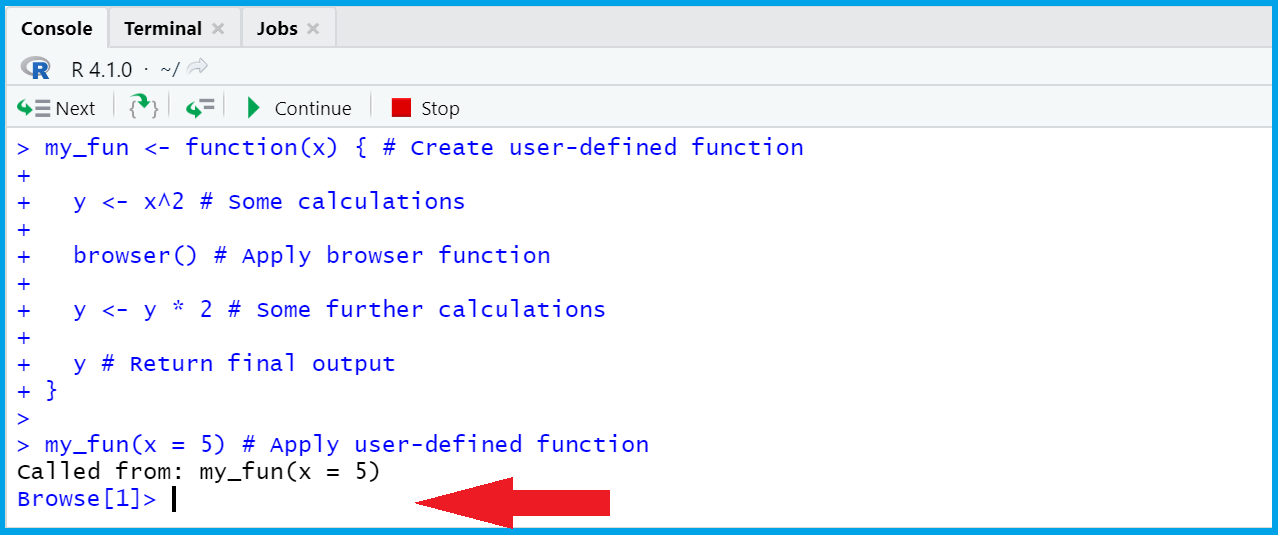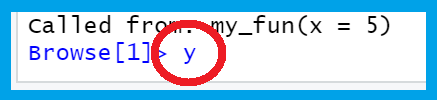# browser Function in R (Example)

This article explains how to inspect the currently used environment using the browser function in the R programming language.

The article will contain one example for the usage of the browser function. To be more precise, the tutorial will consist of this information:

It’s time to dive into the example:

## Example: Applying browser() within User-Defined Function

This section illustrates how to manually create a function that contains a call of the browser function.

Have a look at the following R code:

```my_fun <- function(x) {        # Create user-defined function

y <- x^2                     # Some calculations

browser()                    # Apply browser function

y <- y * 2                   # Some further calculations

y                            # Return final output
}```

The previous R code basically contains five steps:

1. my_fun ＜- function(x) { sets up our user-defined function. At this point, we specify that we want to use a data object called x within the function.
2. y ＜- x^2 performs some calculations with the input data object x and stores the result of these calculations in a new data object called y.
3. browser() provides the user with the possibility to inspect the elements of the function environment.
4. y ＜- y * 2 performs some additional calculations with the new variable y. Note that the previous value that we have calculated in step 2) is overwritten.
5. y returns the final y-value as output from the function.

Enough theoretical explanations – Let’s apply our function!

The following R code applies our user-defined function to the value 5:

`my_fun(x = 5)                  # Apply user-defined function`

After executing the previous R code, the following Browse window appears in the RStudio console:We can use this browser window to inspect the elements that are currently stored in the environment of our function.

For instance, we can print the current y-variable by typing y and Enter as shown below:After pressing enter, the following output is returned:As you can see, the value 25 (i.e. the y-value at this point of the function) is returned.

Next, we can exit the browser mode by typing Esc.

Afterwards, the output at the end of the function (i.e. the final y-value) is returned:

`#  50`

Looks good!

The present example has shown a very basic application of the browser function in R. However, in more complex settings it can be very useful to evaluate and debug your code.

## Video, Further Resources & Summary

If you need more info on the R programming syntax of this tutorial, I recommend watching the following video on my YouTube channel. I’m explaining the topics of this tutorial in the video.

In addition, you may want to have a look at the other articles on this website. Some tutorials that are related to the usage of the browser function are shown below.

Summary: In this tutorial, I have shown how to apply the browser function to interrupt or stop the execution of an expression and allow the inspection of the currently used environment in R.